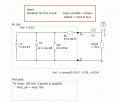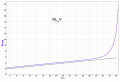# PhotoVoltaic cell Equation: I & V from Rload

#### tonigau

Joined May 2, 2011
14
I need an equation to calculate Vcell & Icell of PhotoVoltaic cell from varying Load Resistance.

In VisualBasic & Excel I have a simplified eqn for Icell from varying Vcell & it works ok. {Icell = CellIsc - (Io1 * (Exp(Vcell / PVt)))}. But I need to Vary Rload & output Icell & Vcell just like circuit simulation.(example attached)
In VB I can calculate Rload from: Vcell/Icell & it correlates (within scope of simplified eqn) to circuit simulation. But I can't get Rload -> I & V equation with success of the non linear part.

I have simulate circuit in qucs for reference & outputs agree to real PV panel data.#### Attachments

• 26.9 KB Views: 7

#### fourtytwo

Joined May 2, 2017
38
I think your question is can I simulate a diode junction in visual basic/xcell and the answer is probably yes but only with extreme difficulty! Given you have a perfectly adequate simulator I cannot imagine why you want to do this.

I would also question your diode model in your simulation as it is normally required to adjust N as well to get a good match, also a connection resistance of just 1.3 milli ohms is rather fancifulI presume you have a hidden parameter somewhere to multiply the Vf of D1 according to the number of cells, it does not appear to be in your postings ?

Last edited:

#### wayneh

Joined Sep 9, 2010
16,400
In VisualBasic & Excel I have a simplified eqn for Icell from varying Vcell & it works ok. {Icell = CellIsc - (Io1 * (Exp(Vcell / PVt)))}.
I may be able to get you a solution, the inverse of the function, if you can give me a cleaner version with all terms defined. I think I get the general idea but details matter.

#### tonigau

Joined May 2, 2011
14
Fortytwo,
Sorry to confuse, the graphic & attached are just for reference & to help explain what I need, yes there are parameters & equations for scaling etc... ( not shown) for full & accurate simulation, it very closely matches my real PV panel with load(s) I have connected (heating element's).
I need to implement this in VB6 because my program interacts with the pic16Fxx simulator .

wayneh,
"the inverse of the function" yes, this sounds promising...

Here is the VB code...

'Inputs:
Io1 = 0.00000000114 ' PV Diode current
PVt = 0.02568 ' Cell temperature variant
Cell_Voc = 0.6216 ' Open circuit cell Volt
Cell_Isc = 8.7800 ' Illumination current (at 1x sun)
Cell_Vmp = 0.5016 '* Cell Volt at MPP
Rs = 0.0055 '* Cell series Resistance (...to be confirmed)
Rp = 10.0e+3 '* Cell parallel Resistance
' (* not in this eqn for now)

'Equation:
' Icell = Cell_Isc - (Io1 * (Exp(Vcell / PVt)))

'Implementation:
Np = 1024
For N = 1 to Np
Vcell = Cell_Voc / Np * N
Icell = Cell_Isc - (Io1 * (Exp(Vcell / PVt)))
Next

Notes: (code formatting got a bit screwed up)
This is extracted from my VB program, Vcell varies linearly from near zero to CellVoc.

This simplified equation does not have Rs, Rp or implement PVt correctly, I will amend this later.
But it does produce output that is close enough with simulated & published PV cell characteristics.

This equation is not for the measured output to Rload of a PV cell, but its operating characteristics of the PV cell which include negative current due to the diode that conducts more as Vcell approaches Cell_Vmp.
Some of the inputs may not be accurate , but should not be concern at this time.

My need is to vary Rload & obtain Icell & Vcell. (Ultimately I would like to get Iout into load (positive current only), but I will worry about that later)

Many thanks

#### wayneh

Joined Sep 9, 2010
16,400
Fortytwo,
Sorry to confuse, the graphic & attached are just for reference & to help explain what I need, yes there are parameters & equations for scaling etc... ( not shown) for full & accurate simulation, it very closely matches my real PV panel with load(s) I have connected (heating element's).
I need to implement this in VB6 because my program interacts with the pic16Fxx simulator .

wayneh,
"the inverse of the function" yes, this sounds promising...

Here is the VB code...

'Inputs:
Io1 = 0.00000000114 ' PV Diode current
PVt = 0.02568 ' Cell temperature variant
Cell_Voc = 0.6216 ' Open circuit cell Volt
Cell_Isc = 8.7800 ' Illumination current (at 1x sun)
Cell_Vmp = 0.5016 '* Cell Volt at MPP
Rs = 0.0055 '* Cell series Resistance (...to be confirmed)
Rp = 10.0e+3 '* Cell parallel Resistance
' (* not in this eqn for now)

'Equation:
' Icell = Cell_Isc - (Io1 * (Exp(Vcell / PVt)))

'Implementation:
Np = 1024
For N = 1 to Np
Vcell = Cell_Voc / Np * N
Icell = Cell_Isc - (Io1 * (Exp(Vcell / PVt)))
Next

Notes: (code formatting got a bit screwed up)
This is extracted from my VB program, Vcell varies linearly from near zero to CellVoc.

This simplified equation does not have Rs, Rp or implement PVt correctly, I will amend this later.
But it does produce output that is close enough with simulated & published PV cell characteristics.

This equation is not for the measured output to Rload of a PV cell, but its operating characteristics of the PV cell which include negative current due to the diode that conducts more as Vcell approaches Cell_Vmp.
Some of the inputs may not be accurate , but should not be concern at this time.

My need is to vary Rload & obtain Icell & Vcell. (Ultimately I would like to get Iout into load (positive current only), but I will worry about that later)

Many thanks
I don’t think there’s an analytical solution but it would be fairly easy to solve this numerically, ie. iteratively. You can do so in Excel or in most programming languages. It takes a little thought and effort to get an algorithm that converges quickly, and to terminate the iterations when sufficient precision has been achieved. In this case I don’t think either will hard.

You make a guess of the voltage across the load. Then you calculate the current that would cause in the load, and calculate the voltage using your equation. Compare the guessed voltage to the calculated one and adjust the voltage guess to be closer to the calculated one. Repeat until the two voltages are close enough.

Last edited:

#### tonigau

Joined May 2, 2011
14
Thanks, I will see if I can implement this method, got me thinking now...

Looking at the graph below, a plot of Rload vs Vout a portion is linear then a curve to max.
I can "trace" visually accurate the curve section with 2 end points & 2 bezier points graphically so I will be able to do this programmatically.
Rload is linear in this graph, so using inverse? of RL_V, the V_RL plot data I should be able to make an algorithm to build array of Vout relative to range of linear Rload values.

Keeping in mind the output is not intended for accurate simulation of PVcell but to close approximate the real operating characteristic for the 2 programs to interact with.